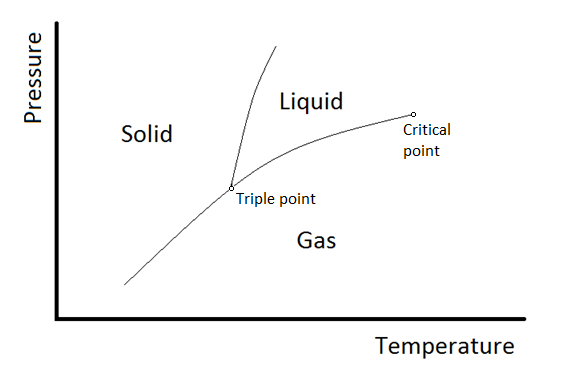Problem: If the phase diagram for a pure substance has two and only two solid phases, how many triple points will it have?A. 0 B. 1 C. 2 D. 3 E. 4

FREE Expert Solution

For this problem, we're being asked to determine how many triple points are present whenever a phase diagram of a pure substance has two solid phases.

Recall that in most phase diagrams, we can distinguish 3 states separated by the lines. Here's an exampleBut there are special cases that a substance can exhibit two solid phases, example is sulfur. The phase diagram of sulfur can be seen below

89% (386 ratings)
Problem Details

If the phase diagram for a pure substance has two and only two solid phases, how many triple points will it have?

A. 0

B. 1

C. 2

D. 3

E. 4